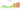# P

## Botswanan Pula

Botswana uses the pula as its currency. It is divided into 100 thebe and has the ISO 4217 code BWP. Given that rain is extremely rare in Botswana, which is home to much of the Kalahari Desert, the word "pula" literally translates as "rain," making it both valuable and fortunate. Additionally, the phrase acts as the nation's slogan. Thebe, or "shield," is a monetary subunit that stands for defense. The public's input was used to choose the names.

#### how has the value of the currency changed in the last year?## USD 1 = BWP 13.522

How much is10 US dollars worth inBotswanan pulas?
At the current exchange rate, 10 US dollars is worth 135.22 Botswanan pulas
How much is50 US dollars worth inBotswanan pulas?
At the current exchange rate, 50 US dollars is worth 676.1 Botswanan pulas
How much is100 US dollars worth inBotswanan pulas?
At the current exchange rate, 100 US dollars is worth 1,352.21 Botswanan pulas
How much is500 US dollars worth inBotswanan pulas?
At the current exchange rate, 500 US dollars is worth 6,761.03 Botswanan pulas
How much is2,000 US dollars worth inBotswanan pulas?
At the current exchange rate, 2,000 US dollars is worth 27,044.12 Botswanan pulas
How much is10 US dollars worth inBotswanan pulas?
At the current exchange rate, 10 US dollars is worth 135.22 Botswanan pulas
How much is50 US dollars worth inBotswanan pulas?
At the current exchange rate, 50 US dollars is worth 676.1 Botswanan pulas
How much is100 US dollars worth inBotswanan pulas?
At the current exchange rate, 100 US dollars is worth 1,352.21 Botswanan pulas
How much is500 US dollars worth inBotswanan pulas?
At the current exchange rate, 500 US dollars is worth 6,761.03 Botswanan pulas
How much is2,000 US dollars worth inBotswanan pulas?
At the current exchange rate, 2,000 US dollars is worth 27,044.12 Botswanan pulas